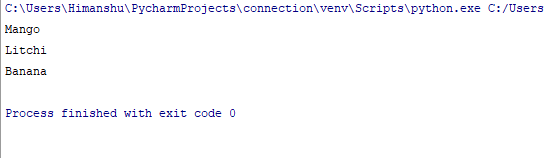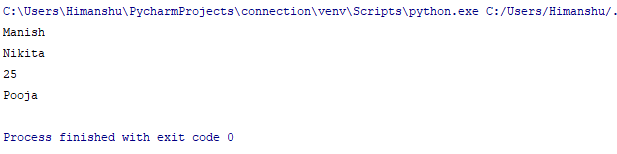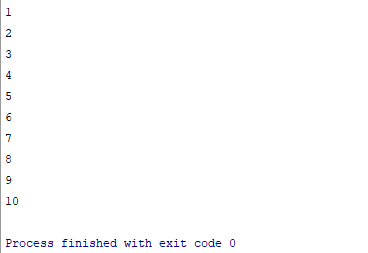×

### Html & Html5

Introduction to Html

Component of Html

Html Form

Html5 basics

Html5 Grid

Html5 Graphics

Html5 Media

Html5 APIs

### Python and Pandas

Introduction to Python

Component of Python

### Python Iterators

Iterator is that object that contains a countable number of values. And through iterator we can transverse through all the values. It is having two types of methods _iter_() and _next_().
Let's understand this by the following program :-

mytuple =("Mango" , "Litchi", "Banana")

fruits = iter(mytuple)

print(next(fruits))

print(next(fruits))

print(next(fruits))

### Output of this ProgramWe can see that we are getting each and every value.

looping through an iterator

We can use for loop in place of iteration . Let’s understand this by the following program :-

list=["Manish", "Nikita", 25 , "Pooja" ]

for x in list:

print(x)

### Output of this ProgramHere in the output we can see that all items we are getting one by one.

stopiteration

If you are having the iteration which will continue forever than to prevent the iteration , we can use the Stopiteration statement.

class Numbers:

def __iter__(self):

self.no = 1

return self

def __next__(self):

if self.no <= 10:

x = self.no

self.no +=1

return x

else:

raise StopIteration

myclass= Numbers()

myiter= iter(myclass)

for x in myiter:

print(x)

### Output of this ProgramSo here through Stopiteration statement we just stop our iteration.

python training insitute| Best IT Training classes in Gurgaon | python training